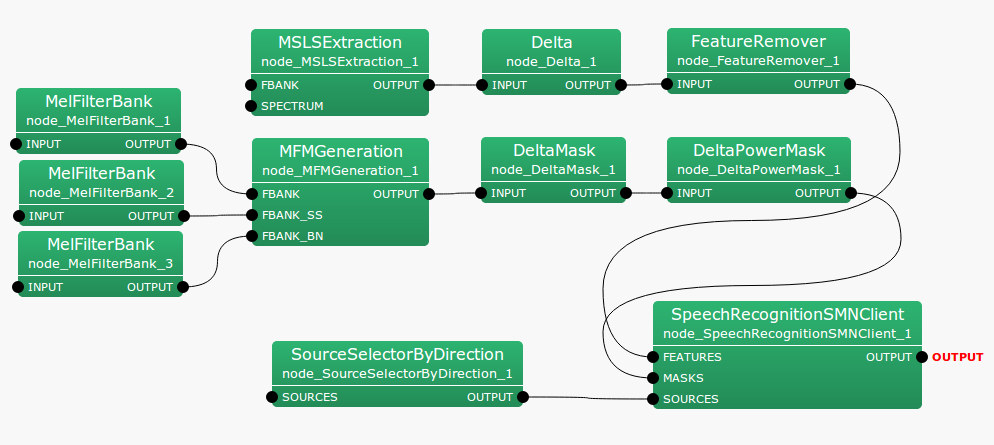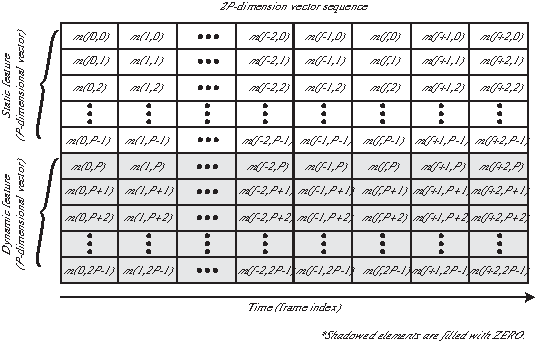6.5.3 MFMGeneration

6.5.3.1 Details of the node

This node generates Missing Feature Masks (MFM) for speech recognition based on missing feature theory.

6.5.3.2 Necessary file

No files are required.

When to use

This node is used for performing speech recognition based on the missing feature theory. MFMGeneration  generates Missing Feature Masks from the outputs of PostFilter  and GHDSS . Therefore, PostFilter  and GHDSS  are used as a prerequisite.

Typical connectionFigure 6.100: Connection example of MFMGeneration

6.5.3.3 Input-output and property of the node

Table 6.87: Parameter list of MFMGeneration
 Parameter name Type Default value Unit Description FBANK_COUNT 13 Dimension number of acoustic feature THRESHOLD 0.2 Threshold value to quantize continuous values between 0.0 and 1.0 to 0.0 (not reliable) or 1.0 (reliable)

Input

FBANK

: Map<int, ObjectRef>  type. A data pair consisting of the sound source ID and a vector of mel filter bank output energy (Vector<float> type) obtained from the output of PostFilter .

FBANK_SS

: Map<int, ObjectRef>  type. A data pair consisting of the sound source ID and a vector of mel filter bank output energy (Vector<float> type) obtained from the output of GHDSS .

FBANK_BN

: Map<int, ObjectRef>  type. A data pair consisting of the sound source ID and a vector of mel filter bank output energy (Vector<float> type) obtained from the output of BGNEstimator .

Output

OUTPUT

: Map<int, ObjectRef>  type. A data pair consisting of the sound source ID and a missing feature vector of type Vector<float> . Vector elements are 0.0 (not reliable) or 1.0 (reliable). The output vector is of dimension 2*FBANK_COUNT, and dimension elements greater than FBANK_COUNT are all 0. These elements are placeholders, which will later store the dynamic information of the Missing Feature Masks.

Parameter

FBANK_COUNT

: int  type. The dimension of acoustic features.

THRESHOLD

: float  type. The threshold value to quantize continuous values between 0.0 (not reliable) and 1.0 (reliable). When setting to 1.0, all features are trusted and it becomes equivalent to normal speech recognition processing.

6.5.3.4 Details of the node

This node generates missing feature masks (MFM) for speech recognition based on the missing feature theory. Threshold processing is performed for the reliability $r(p)$ with the threshold value THRESHOLD and the mask value is quantized to 0.0 (not reliable) or 1.0 (reliable). The reliability is obtained from the output energy $f(p),$ $b(p),$ $g(p),$ of the mel filter bank obtained from the output of PostFilter , GHDSS  and BGNEstimator . Here, the mask vector of the frame number $f$ is expressed as:

 $\displaystyle \boldsymbol {m}(f)$ $\displaystyle =$ $\displaystyle [ m(f,0),m(f,1), \dots ,m(f,P-1)]^ T$ (161) $\displaystyle m(f,p)$ $\displaystyle =$ $\displaystyle \left\{ \begin{array}{ll}1.0, & r(p)>{THRESHOLD} \\ 0.0, & r(p)\leq {THRESHOLD} \\ \end{array} \right. ,$ (162) $\displaystyle r(p)$ $\displaystyle =$ $\displaystyle \min ( 1.0, (f(p)+ 1.4 * b(p))/(fg(p)+ 1.0)),$ (163)

Here, $P$ is the dimension number of the input feature vector and is a positive integer designated in FBANK_COUNT. The dimension number of the vector actually output is 2*FBANK_COUNT. Dimension elements more than FBANK_COUNT are filled up with 0. This is a placeholder for dynamic feature values. Figure 6.101 shows a schematic view of an output vector sequence.Figure 6.101: Output vector sequence of MFMGeneration